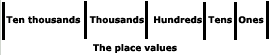Home    |    Teacher    |    Parents    |    Glossary    |    About UsThe idea of place value is at the heart of our number system. First, however, a symbol for nothing--our zero--had to be invented. Zero "holds the place" for a particular value, when no other digit goes in that position. For example, the number "100" in words means one hundred, no tens, and no ones. Without a symbol for nothing, our decimal number system wouldn't work.

Beginning with the ones place at the right, each place value is multiplied by increasing powers of 10. For example, the value of the first place on the right is "one", the value of the place to the left of it is "ten," which is 10 times 1. The place to the left of the tens place is hundreds, which is 10 times 10, and so forth.

For easier readability, commas are used to separate each group of three digits, which is called a period. When a number is written in this form, it is said to be in "standard form."Examples

Numbers can be represented in many ways, but standard form is usually the easiest and shortest way. Here are some numbers expressed in different forms, with their standard form shown alongside. Which form do you think is the best?

Example 1

one billion, sixty million, five hundred twenty thousand

1,060,520,000

Example 2

four hundred sixteen thousand, seven hundred thirty-one

416,731

Example 3

6,000,000 + 70,000 + 20 + 1

6,070,021

Try It!

In each of these numbers, what value does the digit 5 have?

In 17,526,010 the 5 represents " five hundred thousands"
In 2,110,735,000 the 5 represents "five thousands"

 Homework Help | Pre-Algebra | NumbersEmail this page to a friendSearch·  Place value·  Decimal numbers·  Estimating and     rounding·  Adding / subtracting     decimals·  Multiplying decimals·  Dividing decimals·  Percent·  Exponents·  Square roots·  Signed integers·  Adding and     subtracting integers·  Multiplying and     dividing integers·  Properties of integersFirst Glance In Depth Examples WorkoutPlace value